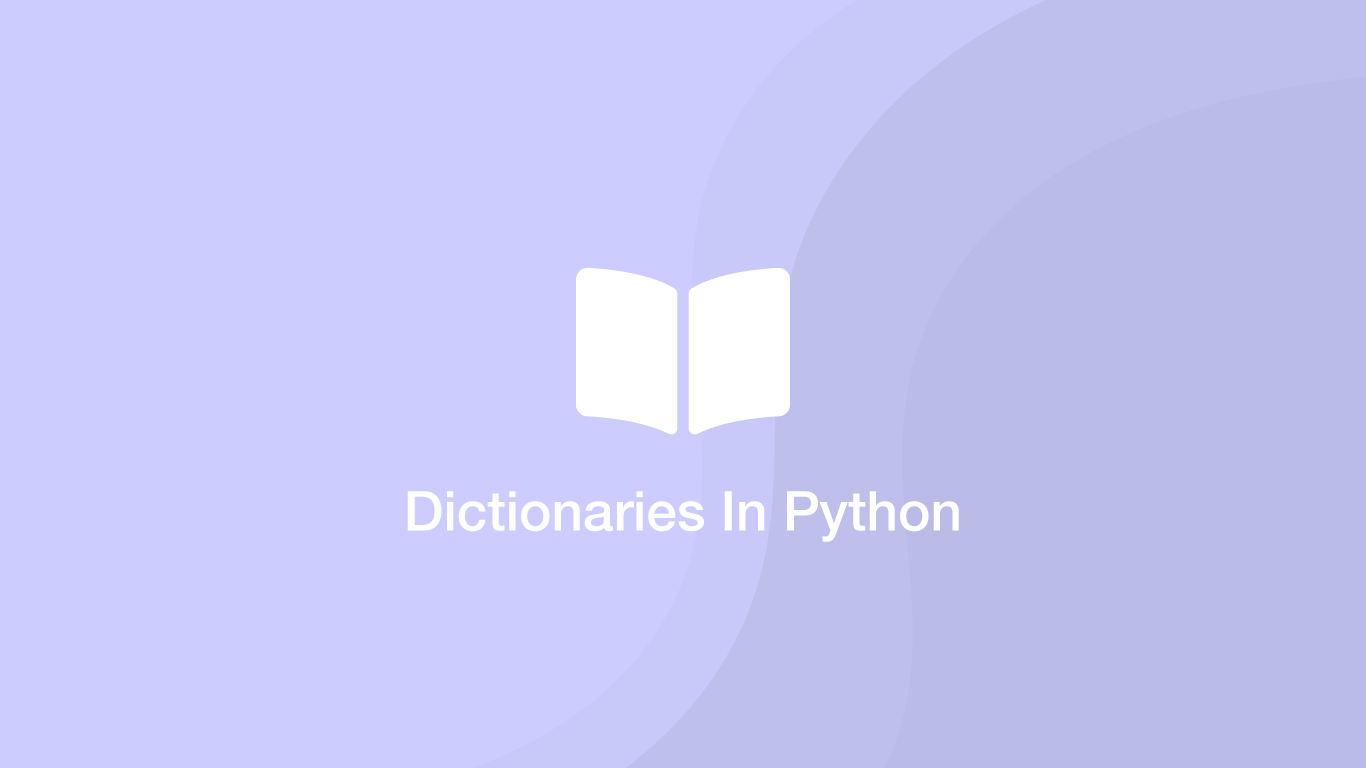# How to Use Dictionaries In PythonDictionaries in Python are used to store data in key-value pairs and are similar to associative arrays in other programming languages like PHP. A Python dictionary is sometimes referred to as a look-up table because the keys can be named something related to the values they are paired with, making them easier to work with.

In this tutorial, we will learn how to use dictionaries in Python using examples.

## Creating a Dictionary

Let's begin by creating an empty dictionary and storing it in a variable called `d`. To create a new dictionary type `{}` (curly brackets).

``````d = {}
``````

A dictionary can also be created with some predefined keys and values. Keys and values are paired with a `:` (colon) and separated by `,` (commas). If you are using string values they will need to be wrapped in `""` (double quotes).

``````d = {"John" : 28, "Jeff" : 25}
``````

## Adding Key-value Pairs to a Dictionary

To add a new key-value pair, place `[]` (square brackets) after the dictionary variable and inside the square brackets type the name of the key wrapped `""` (double quotes). Then type `=` (equals) followed by the value to set.

``````d["Ken"] = 33
``````

## Getting a Value from a Dictionary

To get the value of a dictionary key put the name of the key inside `[]` (square brackets). The example below is printing the data so we can see the output.

``````print(d["John"])
``````
``````28
``````

## Updating the Value in an Existing Key

Updating the value of an existing key is written in exactly the same way as adding a new key-value pair.

``````d = {'John': 28, 'Jeff': 25, 'Ken': 33}

d["John"] = 20

print(d["John"])
``````
``````20
``````

## How to Iterate Over Key-Value Pairs in a Dictionary

We can iterate over key-value pairs in a dictionary using a `for` loop. Check out my article on for loops in Python if you are not familiar with them.

To iterate over both the key and the value, we can put a comma after the iteration variable and use `value in d.items()` .

``````for key, value in d.items():
print(key, value)
``````
``````John 28
Jeff 25
Ken 33
``````

## Conclusion

You now know what Python dictionaries are and how to, create, update and iterate over them.